#Introduction to Angles: Acute, Obtuse, Straight, and Right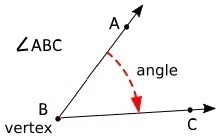An angle is the amount of turn between two lines around their common point (the vertex). In the angle shown above, endpoint B is the vertex. The name of an angle uses an angle symbol followed by three letters. The letter from one side, the letter from the other side, and the letter of the vertex. The letter of the vertex must go in the middle.

Or alternatively, an angle can be identified by giving it a single letter name. The angle shown above might be named angle X.

Measuring Angles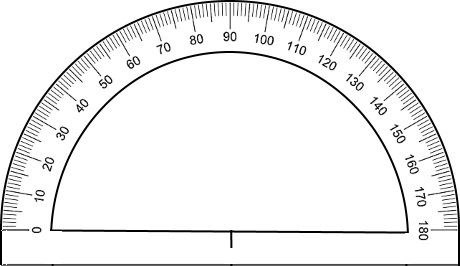The unit of measure for an angle is the degree. A full rotation around the vertex is 360 degrees. An angle can be measured using a protractor.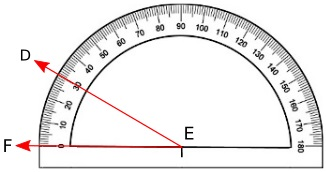To use a protractor, locate the mark at the halfway point on the inside of the straight side of the protractor. Place that mark over the vertex of the angle. Place one of the sides of the angle along the inside of the straight side of the protractor. Read the number where the other side of the angle intersects the protractor. That number is the angle. The angle shown above measures 30 degrees on the protractor.

Classifying Angles

Angles an be classified based upon the size of the angle. Angle types are acute, right, obtuse, straight, and reflex.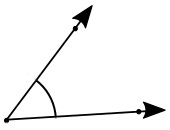An acute angle is but more than 0 degrees, and less than 90 degrees.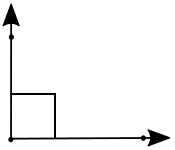A right angle is exactly 90 degrees. Since right angles are so common and important in geometry, instead of a small arc, the angle is marked with a small square and the measure in degrees is usually omitted.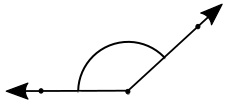An obtuse angle is greater than 90 degrees and less than 180 degrees.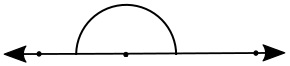A straight angle is exactly 180 degrees. A 180 degree angle results in the sides of the angle pointing in exactly opposite directions, forming a single straight line through the vertex of the angle.A reflex angle is greater than 180 degrees and less than 360 degrees.